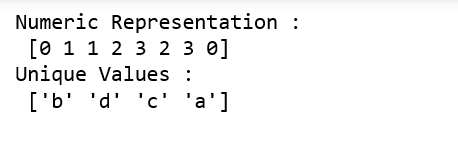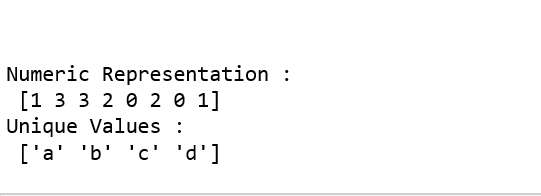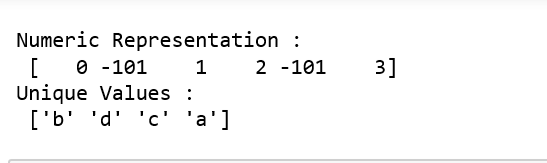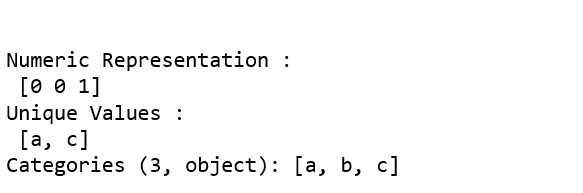Related Articles

# Python | Pandas.factorize()

• Last Updated : 27 Sep, 2018

pandas.factorize() method helps to get the numeric representation of an array by identifying distinct values. This method is available as both `pandas.factorize()` and `Series.factorize()`.

Parameters:
values : 1D sequence.
sort : [bool, Default is False] Sort uniques and shuffle labels.
na_sentinel : [ int, default -1] Missing Values to mark ‘not found’.

Return: Numeric representation of array

Code: Explaining the working of factorize() method

 `# importing libraries``import` `numpy as np``import` `pandas as pd``from` `pandas.api.types ``import` `CategoricalDtype`` ` `labels, uniques ``=` `pd.factorize([``'b'``, ``'d'``, ``'d'``, ``'c'``, ``'a'``, ``'c'``, ``'a'``, ``'b'``])`` ` `print``(``"Numeric Representation : \n"``, labels)``print``(``"Unique Values : \n"``, uniques)``# sorting the numerics``label1, unique1 ``=` `pd.factorize([``'b'``, ``'d'``, ``'d'``, ``'c'``, ``'a'``, ``'c'``, ``'a'``, ``'b'``], ``                                                           ``sort ``=` `True``)`` ` `print``(``"\n\nNumeric Representation : \n"``, label1)``print``(``"Unique Values : \n"``, unique1)``# Missing values indicated``label2, unique2 ``=` `pd.factorize([``'b'``, ``None``, ``'d'``, ``'c'``, ``None``, ``'a'``, ], ``                                              ``na_sentinel ``=` `-``101``)`` ` `print``(``"\n\nNumeric Representation : \n"``, label2)``print``(``"Unique Values : \n"``, unique2)``# When factorizing pandas object; unique will differ ``a ``=` `pd.Categorical([``'a'``, ``'a'``, ``'c'``], categories ``=``[``'a'``, ``'b'``, ``'c'``])`` ` `label3, unique3 ``=` `pd.factorize(a)`` ` `print``(``"\n\nNumeric Representation : \n"``, label3)``print``(``"Unique Values : \n"``, unique3)`Attention geek! Strengthen your foundations with the Python Programming Foundation Course and learn the basics.

To begin with, your interview preparations Enhance your Data Structures concepts with the Python DS Course. And to begin with your Machine Learning Journey, join the Machine Learning – Basic Level Course

My Personal Notes arrow_drop_up